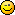###Author Topic: Rounding numbers!  (Read 2488 times)

####Ozden79

• Mr. Polyvector
•• Posts: 121##### Rounding numbers!
« on: 2010-Jan-29 »
In some cases, I need to round a number to closest upper and some cases into closest lower integer value. I only see INTEGER command which I think only rounds to closest lower integer number. Is there any such command in GLBasic or do I need to implement my own?

Thanks,

Ãƒâ€“zden

####Quentin

• Prof. Inline
•• Posts: 915##### Re: Rounding numbers!
« Reply #1 on: 2010-Jan-29 »
if you want to round it business like, you can try this (I think it was already ask somewhere though)

Code: (glbasic) [Select]
`PRINT round(5.434344), 0, 0PRINT round(5.66344), 0, 20SHOWSCREENKEYWAIT// ------------------------------------------------------------- //// ---  ROUND%  ---// ------------------------------------------------------------- //FUNCTION round%: p_num RETURN INTEGER(p_num + .5)ENDFUNCTION // ROUND%`

####Kitty Hello

• code monkey
• Prof. Inline
••• Posts: 10851
• here on my island the sea says 'hello'##### Re: Rounding numbers!
« Reply #2 on: 2010-Feb-01 »
bad for negative numbersYou could round like this:
Code: (glbasic) [Select]
`FUNCTION Round: number, precLOCAL mulf = MAX(POW(10., prec), 1e-12) number = number *mulfLOCAL ret IF number<0 RETURN INTEGER(number-.5)/mulf ENDIF RETURN INTEGER(number+.5)/mulfENDFUNCTION`
But usually you only want rounded numbers for output. Then use FORMAT\$().

####Qedo

• Dr. Type
••• Posts: 382
• to program what I have do how should programming?##### Re: Rounding numbers!
« Reply #3 on: 2020-Apr-05 »
Good for positive and negative numbers

FUNCTION round%: p_num
RETURN INTEGER(p_num+(SGN(p_num)*0.5))
ENDFUNCTION // ROUND%